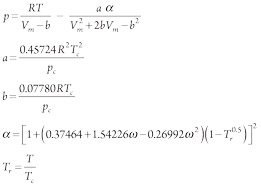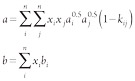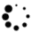# Accurate prediction of phase equilibrium properties—Part 1

Phase equilibrium properties, such as bubble-point, hydrocarbon dewpoint, water dewpoint, phase envelope, two-phase compositions, compressibility factor, hydrate equilibrium properties, etc., for hydrocarbon mixtures have been calculated accurately using the Peng-Robinson cubic equation of state by implementing constant and temperature-dependent binary interaction parameters in the Van der Waals mixing rule.

Phase equilibrium properties, such as bubble-point, hydrocarbon dewpoint, water dewpoint, phase envelope, two-phase compositions, compressibility factor, hydrate equilibrium properties, etc., for hydrocarbon mixtures have been calculated accurately using the Peng-Robinson cubic equation of state by implementing constant and temperature-dependent binary interaction parameters in the Van der Waals mixing rule.

These phase equilibrium properties find usage in the design and operation of all kinds of hydrocarbon equipment, such as hydrocarbon pipelines, hydrocarbon pumps, pressure vessels, hydrocarbon storages and distillation columns. Phase equilibrium properties are also required in upstream oil and gas activities like well simulation and oil and natural gas production. Atmospheres of various heavenly bodies are made of hydrocarbons, such as methane (CH4), ethane (C2H6), propane (C3H8), etc., and inorganic gases like nitrogen (N), carbon dioxide (CO2) and hydrogen sulfide (H2S). Simulation of these atmospheres also requires knowledge of phase equilibrium properties.

A new vapor liquid equilibrium (VLE) softwarea has been developed using basic calculation tools like MS Excel and Visual Basic for Applications (VBA, macro programming) to accurately calculate various phase equilibrium properties. The Peng-Robinson cubic equation of state and Van der Waals mixing rule using a combination of temperature-dependent and constant binary interaction parameters are used for these calculations. The proprietary VLE software is designed to give accurate phase equilibrium properties for any mixture of nonpolar or mildly polar hydrocarbons and inorganic gases up to a maximum number of 112 components in the hydrocarbon mixture.

## Theory

Accurate prediction of phase equilibrium properties requires solving models using complex equations. The cubic equations of state-based models are preferred over other models, as these models require the same set of equations to be solved for both liquid and vapor phase. Also, cubic equations of state require few parameters, such as critical temperature, critical pressure and acentric factor, to calculate various phase equilibrium properties. These parameters are well-documented and readily available for most hydrocarbons. Cubic equations of state give accurate results for non-polar and mildly polar hydrocarbons and their mixtures. Since petroleum products are mixtures of predominantly non-polar and mildly polar hydrocarbons, cubic equations of state are widely used in predicting phase equilibrium properties in the oil and natural gas industries.

Numerous cubic equations of state are given in literature. Of these, the Soave-Redlich-Kwong cubic equation of state and the Peng-Robinson cubic equation of state are the oldest and most widely used. Generally, the Peng-Robinson cubic equation of state is preferred over the Soave-Redlich-Kwong cubic equation of state, as it can more accurately predict liquid phase properties.1,2

The Peng-Robinson cubic equation of state was developed in 1976 at the University of Alberta by Deng-Yu Peng and Donald Robinson; various variations of the Peng-Robinson cubic equation of state have been proposed since its inception. The basic form of the Peng-Robinson cubic equation of state (as proposed in 1976) is given in Eq.1:(1)

In polynomial form (Eq. 2):(2)

where w is the acentric factor of the species, and R is the universal gas constant.3

For pure hydrocarbons, Eqs. 1 and 2 can be used as they are. For calculations involving hydrocarbon mixtures, various types of mixing rules are proposed. Most of these mixing rules are complex and difficult to implement, and some require parameters that can be calculated only experimentally. The Van der Waals mixing rule is the simplest mixing rule used in the Peng-Robinson equation requiring the minimum number of parameters that can be calculated theoretically. Mixture parameters for the Van der Waals mixing rule are calculated using Eq. 3:(3)

where: a and b = the interaction energy and molecular size parameters for the mixture

ai, bi = a and b parameters for component i in the mixture

xi = composition (mole fraction) for component i in the mixture

kij = binary interaction parameter

n = number of components in the mixture.

## Interaction parameters

Binary interaction parameters are used to tune the Peng-Robinson cubic equation of state to achieve accurate results of phase equilibrium properties. Binary interaction parameters can be calculated experimentally for pairs of hydrocarbons. To avoid cumbersome experiments to find binary interaction parameters for different pairs of hydrocarbons, various correlations are proposed in literature to theoretically calculate those binary interaction parameters based on known properties of hydrocarbons included in the pair.4

Various chemical engineering software packages are available in the market to determine phase equilibrium properties for hydrocarbon mixtures. While these software packages use different types of binary interaction parameters to tune their results, most have limitations on the total number of components that can be included in the hydrocarbon mixtures for which the phase equilibrium properties are to be calculated. Also, in various cases, calculation of phase equilibrium properties for off-beat compositions of hydrocarbon mixtures is required.

Most of these chemical engineering software packages cannot calculate phase equilibrium properties for off-beat compositions of hydrocarbon mixtures, even within the limitation of the number of components permissible in the hydrocarbon mixtures. Additionally, as water is a highly polar compound and the Peng-Robinson cubic equation of state can give only accurate results of phase equilibrium properties for nonpolar or mildly polar hydrocarbons and inorganic gases and their mixtures, more complex thermodynamics equations are used to calculate phase equilibrium properties of mixtures of hydrocarbons in which water is also present as a component. These complex thermodynamics equations require complex calculations and require parameters that can be calculated only experimentally. This makes these equations unsuitable for the calculation of phase equilibrium properties like water dewpoint and hydrate equilibrium properties for complex mixtures of hydrocarbons and water containing several components.

A new chemical engineering phase equilibrium softwarea has been developed and uses the Peng-Robinson cubic equation of state to calculate various phase equilibrium properties of hydrocarbon mixtures. The proprietary software can calculate the following phase equilibrium properties:

• Bubble-point temperature values at a given pressure
• Bubble-point pressure values at a given temperature
• Hydrocarbon dewpoint temperature values at a given pressure
• Hydrocarbon dewpoint pressure values at a given temperature
• Phase envelope curve
• Phase envelope curve with CO2 freezing and frost curve
• Two-phase composition inside phase envelope
• Water dewpoint curve
• Gas hydrate equilibrium curve
• Gas hydrate-based gas separation calculation
• Liquid compressibility factor values
• Vapor compressibility factor values
• Binary distillation column calculation curves
• Binary/multi-component distillation column stage-by-stage calculations
• Thermodynamic properties
• Phase equilibrium calculations with pseudo components
• Phase equilibrium calculations for mixtures containing ammonia (NH3).

## Calculating phase equilibrium properties

The software can theoretically calculate the values of binary interaction parameters using a correlation based on critical volumes of the hydrocarbons included in the pair of hydrocarbons for which the binary interaction parameter is being calculated. It uses these values of binary interaction parameters in the Van der Waals mixing rule to calculate phase equilibrium properties. The correlation used to calculate binary interaction parameters in the phase equilibrium software is given in Eq. 4:(4)

where vci and vcj are critical volumes of i and j components, and n is the exponent that is taken to be equal to 1.2, but its value can be varied to tune the hydrocarbon dewpoint results, as per the experimentally determined values of hydrocarbon dewpoint for the hydrocarbon mixture.

However, these binary interaction parameter values will not yield correct results if one of the components in the pair of components being calculated is water. As mentioned, more complex equations than the Peng-Robinson cubic equation of state are used to calculate phase equilibrium properties like the water dewpoint curve and gas hydrate equilibrium curves when hydrocarbon mixtures exist with water. To use the Peng-Robinson cubic equation of state and Van der Waals mixing rule to calculate such mixtures, the phase equilibrium software uses temperature-dependent binary interaction parameters in the Van der Waals mixing rule.

## E-PPR78

These temperature-dependent binary interaction parameters are calculated using the Enhanced Predictive Peng-Robinson 78 (E-PPR78) equation, which uses a modified form of the Peng-Robinson cubic equation of state presented in 1978. The E-PPR78 equation gives a predictive correlation to calculate temperature-dependent binary interaction parameters using group contribution. Besides pairs of components including water, the E-PPR78 equation can be used to give predictive values of temperature-dependent binary interaction parameters for pairs of components including paraffins, cyclo-paraffins, olefins, diolefins, alkynes, aromatics, thiols and inorganic gases such as nitrogen, CO2, H2S, hydrogen, argon and oxygen, giving more accurate results than constant binary interaction parameters or when no binary interaction parameters are used.

The correlation used for calculating predictive values of temperature-dependent binary interaction parameters as per the E-PPR78 equation using group contribution is given in Eq. 5:(5)

where αik is the fraction of molecule i occupied by group k (occurrence of group k in molecule i divided by the total number of groups present in molecule i). Akl = Alk and Bkl = Blk (where k and l are two different groups) are constant parameters shown in TABLE 1 (Akk = Bkk = 0).5

The phase equilibrium software is a complex combination of macros of MS Excel. Different calculation modules of the software are designed to find different phase equilibrium properties, such as bubble-point temperatures, bubble-point pressures, hydrocarbon dewpoint temperatures, hydrocarbon dewpoint pressures, phase envelope, two-phase compositions, and liquid and vapor compressibility factor values. The phase envelope generated using the software consists of equilibrium temperature values at a specified interval of 0.4 bar and clearly marks the critical point, cricondenbar and cricondentherm for the hydrocarbon mixture.

Apart from equilibrium temperature values at intervals of 0.4 bar, bubble-point and dewpoint compositions at intervals of 0.4 bar for a hydrocarbon mixture are also determined by the phase equilibrium software, which calculates two values of bubble-point temperature or hydrocarbon dewpoint temperature existing above the critical point of a hydrocarbon mixture (FIG. 1 and TABLE 2). The proprietary software can accurately predict and calculate bubble-point temperature values and hydrocarbon dewpoint temperature values for hydrocarbon mixtures containing high concentration of non-condensables, where no bubble-point temperature values exist for some pressure values or where more than two bubble-point temperature values or more than two hydrocarbon dewpoint temperature values exist for some pressure values (FIG. 2 and TABLE 3). The phase equilibrium software can also calculate accurate two-phase compositions in a retrograde region.FIG. 1. Phase envelope of a typical natural gas mixture using the phase equilibrium software.aFIG. 2. Phase transition curve for a hydrocarbon mixture with a high concentration of non-condensable components using the phase equilibrium software.a

For a hydrocarbon mixture, up to a maximum of 250 × 250 matrix of liquid compressibility factor values, or vapor compressibility factor values at different temperature and pressure values, can be calculated using the phase equilibrium software. These compressibility factor values can be used in other calculations involving the same hydrocarbon mixture.

CO2 freezing and frost curves are required for finding safe operating temperature and pressure conditions for hydrocarbon mixtures containing CO2 at cryogenic conditions. The phase equilibrium software can calculate accurate CO2 freezing and frost curves for any mixture of non-polar or mildly polar hydrocarbons and inorganic gases containing CO2 using temperature-dependent binary interaction parameters, as per E-PPR78. CO2 freezing and frost curves calculated using the software include equilibrium temperature values, fraction of CO2 solidified and equilibrium compositions at intervals of 0.4 bar (FIG. 3 and TABLE 4).FIG. 3. Phase envelope curve with CO2 freezing curve and CO2 frost curve for a CH4-CO2 mixture using the phase equilibrium software.a

Temperature-dependent binary interaction parameters as per E-PPR78 are used in the phase equilibrium software to calculate water dewpoint curves for any mixture of nonpolar or mildly polar hydrocarbons and inorganic gases existing with water. Water dewpoint curves include equilibrium temperature values at intervals of 0.4 bar, starting from 0.4 bar and ending at 800 bar.

Part 2 of this article will appear in the November issue. HP

NOTES

a EQ-COMP vapor liquid equilibrium (VLE) software

LITERATURE CITED

1. Smith, J. M., H. C. Van Ness and M. M. Abbott, Introduction to Chemical Engineering Thermodynamics, 7th Ed., McGraw-Hill, February 2005.
2. Poling, B. E., J. M. Prausnitz and J. P. O’Connell, The Properties of Gases and Liquids, 5th Ed., McGraw-Hill, 2001.
3. Peng, D. Y. and D. B. Robinson, “A new two-constant equation of state,” Industrial & Engineering Chemistry Fundamentals, February 1976.
4. Campbell, J. M., “How to tune the EOS in your process simulation software?” PetroSkills, September 2009, online: http://www.jmcampbell.com/tip-of-the-month/2009/09/how-to-tune-the-eos-in-your-process-simulation-software/
5. Xo, X., J.-N. Jaubert, R. Privat and P. Arpentinier, “Prediction of thermodynamic properties of alkyne-containing mixtures with the E-PPR78 model,” Industrial & Engineering Chemistry Research, June 2017.

## From the Archive